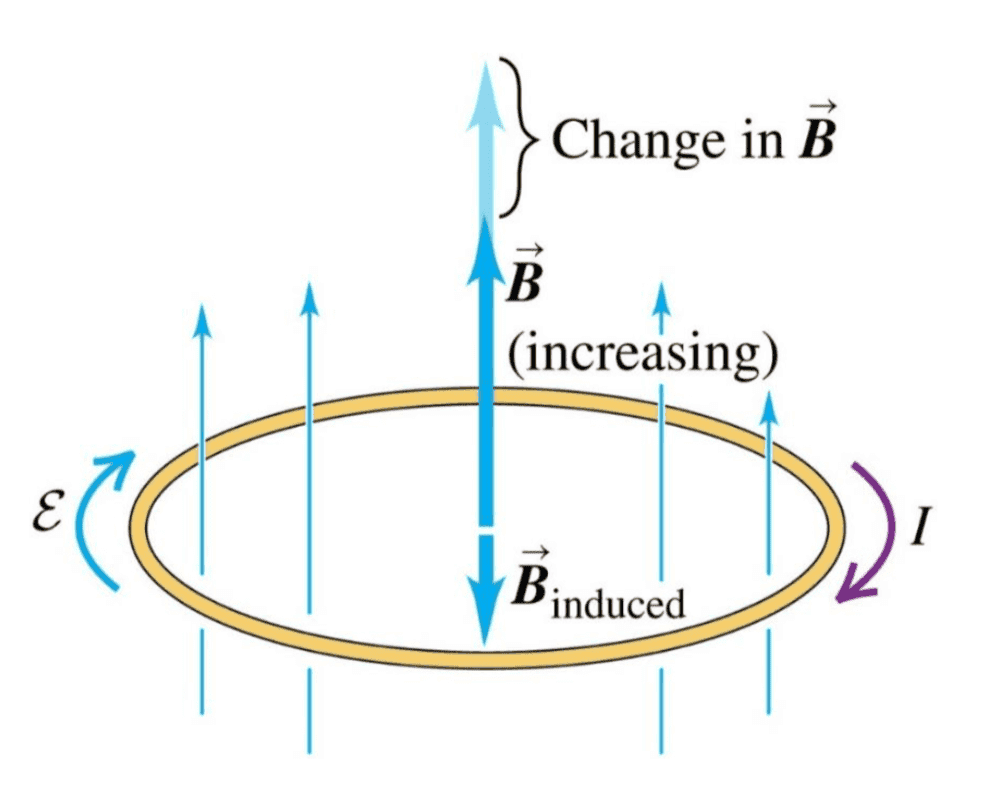# Understand Faraday's Law and Lenz' Law

christang_1023
Homework Statement:
1. Is Lenz's law applied only when there is a closed loop?
2. When a ring in a changing magnetic field is not complete (i.e. open circuit with a small gap), how to analyze the emf of the ring?
Relevant Equations:
Shown belowAbove is an example figure.

2. When a ring in a changing magnetic field is not complete (i.e. open circuit with a small gap), how to analyze the emf of the ring?
According to the general form of Faraday's law, ## \oint \vec{E} \cdot d \vec{s} = -\frac{d \Phi}{dt} ##, I deduce that although it is not a closed loop, there is still emf within the incomplete ring conductor. How can I derive the emf of this ring?

Mentor
The presence or absence of a gap does not affect the induced emf around the ring. It does affect whether there is a current in the ring.

•christang_1023
Homework Helper
Gold Member
Problem Statement
1. Is Lenz's law applied only when there is a closed loop?
2. When a ring in a changing magnetic field is not complete (i.e. open circuit with a small gap), how to analyze the emf of the ring?
Relevant Equations
Shown below

View attachment 242551
Above is an example figure.

2. When a ring in a changing magnetic field is not complete (i.e. open circuit with a small gap), how to analyze the emf of the ring?
According to the general form of Faraday's law, ## \oint \vec{E} \cdot d \vec{s} = -\frac{d \Phi}{dt} ##, I deduce that although it is not a closed loop, there is still emf within the incomplete ring conductor. How can I derive the emf of this ring?
As you say, for a closed, finite-R ring the E field and emf (- to +) are in same direction as the current, which enables you to determine the direction of the emf by Lenz's law.

For a non-existing ring the emf is still in the same direction so you can pretend the ring is solid, the use Lez's law.

The emf is ∫E dl, the integral of the E field over the ring perimeter. If the E field were electrostatic (as in a resistor in a battery-resistor circuit) the potential difference or voltage (- to +) would be the NEGATIVE of ∫E dl.

•christang_1023
Mentor
The presence or absence of a gap does not affect the induced emf around the ring.
Note that here, "induced emf" refers specifically to the emf that is induced by the changing B field via Faraday's Law. Depending on the situation, this might not be the only emf around the ring.